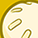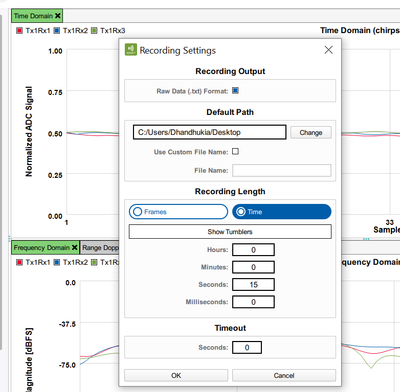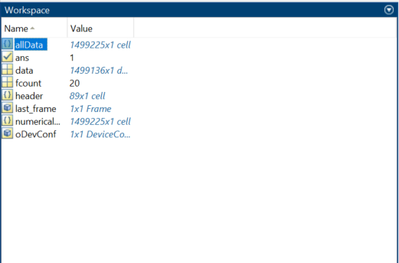Level 1Level 1

# view recorded radar data in matlab

Hey

Is there a way to view the recorded radar data captured in the "Radar Fusion GUI" in matlab signal analyzer app?

They are saved as a .json and raw.bin files which matlab can't read

thanks 🙂ModeratorModerator

# Re: view recorded radar data in matlab

Hello @Taylor70 ,

While recording the data from Radar Fusion GUI, select the Raw Data(.txt) format from recording settings.Following the recording, extract the data from txt file into MATLAB using the following code:

allData = textread('BGT60TR13C_record_20220801-104524.raw.txt', '%s', 'delimiter', '\n');
% Make allData cells empty if numerical
numericalArray = cellfun(@(s) sscanf(s,'%f').' ,allData, 'un', 0);
% Get Data
data = vertcat(numericalArray{:});
plot(data)

Following the execution of the code, save the data variable in the workspace into .mat format to plot it in signal analyzerHope this helps!

Best Regards,

Honey